### Home > A2C > Chapter 10 > Lesson 10.1.1 > Problem10-23

10-23.
1. Convert the following angle measurements from degrees to radians. Homework Help ✎

1. 30°

2. 15°

3. −75°

4. 630°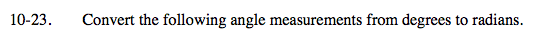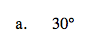$30\degree\cdot\frac{\pi}{180\degree}=?$

$\frac{\pi}{6}$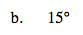See part (a).

$\frac{\pi}{12}$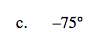See part (a).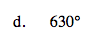See part (a).